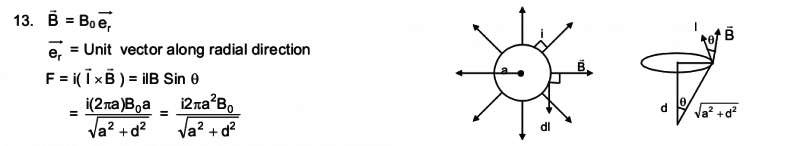# Force on a circular loop due to hypothetical magnetic field.

## Homework Statement

A hypothetical magnetic field existing in a region is given by ##\vec{B}=B_o\vec{e}_r##, where ##\vec{e}_r## denotes the unit vector along the radial direction. A circular loop of radius a, carrying a current ##i##, is placed with its plane parallel to the X-Y plane and the centre at ##(0,0,d)##. Find the magnitude of the magnetic force acting on the loop.

## Homework Equations

Force on a conductor due to a magnetic field : ##\vec{F}_{magnetic}= i\vec{l}##x##\vec{B}##.

## The Attempt at a Solution

A (so far) reliable solution manual (created independent to the referred textbook) plainly explains that as ##\vec{F}_{magnetic}= i\vec{l}##x##\vec{B}## => ##\vec{F}_{magnetic}= i2\pi aB_o(\frac{a}{\sqrt[]{a^2+d^2}})##

Though the final answer is correct, isn't it coincidental and isn't the solution theoretically wrong?

When I've done the problem I think the magnetic field and the length vector are always perpendicular to each other as long as the center of the circular loop is along the z-axis (in the above case) and the magnetic field is radial. So when I resolve the forces I find that component vectors of all the forces acting on the loop cancels out (i.e ##Fcos\theta## around the loop cancels out) whereas the component ## Fsin\theta## adds up and hence the final answer gives : ##\vec{F}_{magnetic}= i2\pi aB_o(\frac{a}{\sqrt[]{a^2+d^2}})##

Am I right? Or am I making a terrible theoretical mistake? Or is the solution manual making a mistake?

Last edited:

BvU
Homework Helper
Don't understand what x and y are. If you mean a and d then I can agree.

Don't see a difference between your answer and that from the solutions manual.... As far as I can reconstruct, you agree and are right. Both.

Don't understand what x and y are. If you mean a and d then I can agree.

Oops! That's supposed to be 'a' and 'd'. Sorry!

Don't see a difference between your answer and that from the solutions manual.... As far as I can reconstruct, you agree and are right. Both.

No! Though the final answers are the same. My theory does not agree with the one given in the solution manual.
The solution manual argues that the force ##|\vec{F}_{magnetic}|= i|\vec{l}||\vec{B}|sin\theta##. This equation says that the angle between the magnetic field and the 'length vector' (which is always in the direction of the current) is ##\theta##.

But my argument as I have said above is : "I think the magnetic field and the length vector are always perpendicular to each other as long as the center of the circular loop is along the z-axis (in the above case) and the magnetic field is radial. So when I resolve the forces I find that component vectors of all the forces acting on the loop cancels out (i.e ##Fcos\theta## around the loop cancels out) whereas the component ## Fsin\theta## adds up and hence the final answer gives : ##\vec{F}_{magnetic}= i2\pi aB_o(\frac{a}{\sqrt[]{a^2+d^2}})##" //repeated

Well comparing equation by equation both are completely similar. But the theoretical side differs.

Last edited:
mfb
Mentor
I guess the solution manual considers the radial component (in the XY plane) of the B-field only. If it does not add an additional explanation, it is a bit too short for a proper solution.

•Hijaz Aslam
Here's the solution given by the solution manual. The diagrams are unreliable.BvU
Homework Helper
I see, and I think the solution manual is indeed a bit slopply with the diagrams and it also carries out the integration dl = a dφ to yield 2πa without mentioning that, thus adding to the confusion.

In short: you are right and you have a good understanding of this exercise. on to the next one :)

•Hijaz Aslam
BvU- Thanks a lot.

BvU